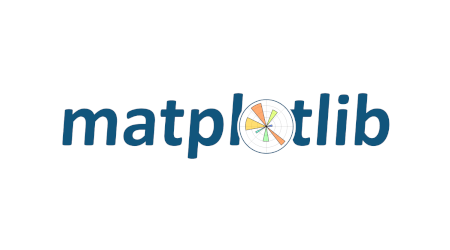## 31 Dec Matplotlib Tutorial

Matplotlib is an open-source plotting library developed by John D. Hunter. Create interactive visualizations in Python with Matplotlib. It is built on NumPy and is one of the most popular libraries for data visualization in Python.

In this tutorial, we will learn how to perform plotting with Python. Visualizations are far better than textual data. Using matplotlib, we can easily create graphs, histograms, bar graphs, etc.

## Python Matplotlib Tutorial Index

If you liked the tutorial, spread the word and share the link and our website Studyopedia with others.